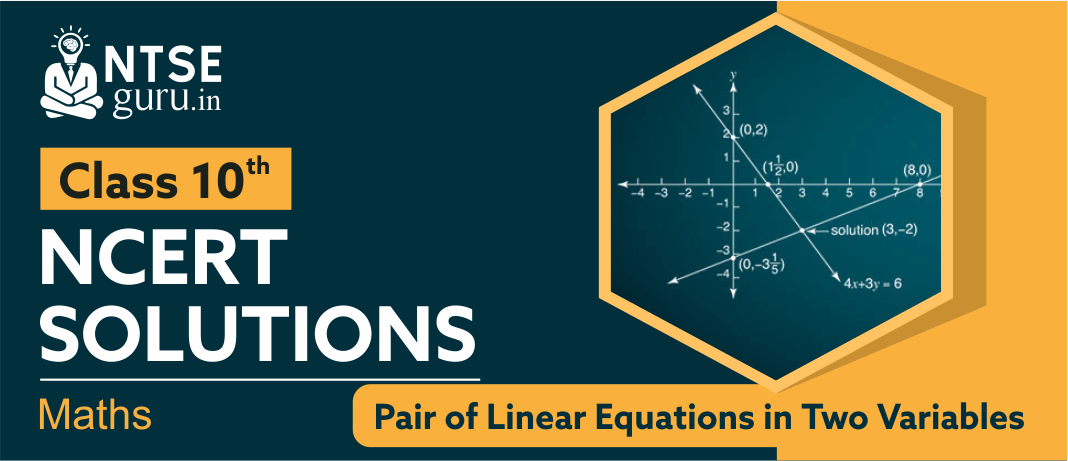# NCERT Solutions For Class 10 Maths Chapter 3NCERT Solutions for Class 10 Maths Chapter 3, ‘Pair of Linear Equations in Two Variables’ explains to students how questions are solved using this concept. If a, b, and r are real numbers (and if a and b are not both equal to 0) then ax+by = r is called a linear equation in two variables.
For example, 3x + 2y = 8 is a linear equation in two variables. A solution of such an equation is an ordered pair of numbers (x, y) that makes the equation true when the values of x and y are substituted into the equation. For example, both (2, 1) and (0, 4) are solutions of the equation but (2, 0) is not a solution.

## Pair of Linear Equations in Two Variables - Class 10 NCERT Solutions

Given below are ‘Pair of Linear Equations in Two Variables - Class 10 NCERT Solutions’ for your reference. These solutions will help you a lot from an exam point of view.

In addition to the above solutions, students are also advised to follow sample papers, previous years solved papers, and practice sets. All this material can be accessed from our website www.ntseguru.in or from our free mobile app NTSE Guru.